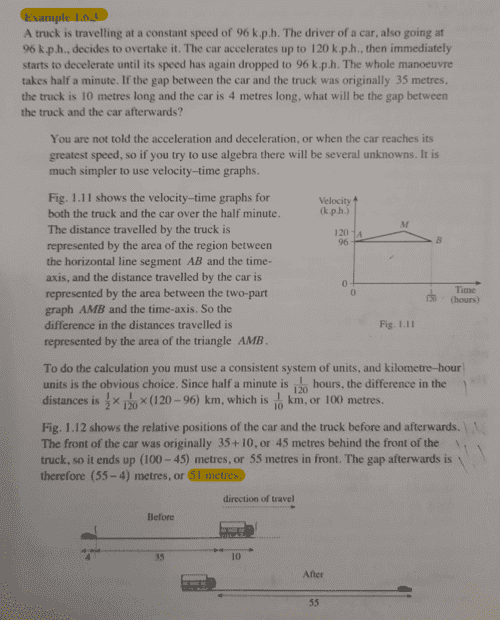# Find the distance between the car and the truck- Mechanics

Gold Member
Homework Statement:
See attached
Relevant Equations:
##s=vt##
Find the problem and the solution below;Find my approach to the problem.
Considering the motion of the car;
##v=u+at##
##33.333= 26.6667+30a##
##a=0.2222##
Therefore it follows that,
##s##=## ut##+##\frac {1}{2}####at^2##
##s##=##(26.67 ×30 +(0.5×0.222×30^2)##
##s=900##metres

The distance that car has to cover in order to overtake the truck is given by
##s= 35+10+4=49##metres

Therefore we shall have, ##900-49= 851## metres

Now considering the distance covered by the truck in ##30## seconds, we shall have
##s=vt##
##s=26.67×30=800.1##metres

Therefore the gap between the car and the truck will be ##851-800.1=51.1##metres.

Any other approach highly appreciated.

Last edited:
•FactChecker

Homework Helper
Gold Member
This is an interesting problem. I would have bet that the rates of acceleration and deceleration would change the answer, even given the restriction that the entire maneuver took half a minute. But I see from their solution that it does not because the horizontal position of point M does not change the area of the triangle AMB.

•chwala and hmmm27
ergospherical
It would simplify the arithmetic slightly to work in the rest frame of the truck i.e. subtract ##96 \mathrm{kph}## from all of the velocities.

Homework Helper
@chwala, a couple of points.

First, you assume that the acceleration is constant over the half minute (and the deceleration instantaneous). You are not told that. As FactChecker pointed out, it turns out that the rates of acceleration and deceleration don't make a difference, but it's not obvious that you knew that and it's not clear from your working (and it was not obvious to me or FactChecker either). The geometric method, on the other hand, makes it clear.

Second, you once again unnecessarily convert to m/s. your final answer of 51.1 m is slightly erroneous, not because of a mistake in the conversion, but because of premature rounding off. You say
s = 26.67*30 = 800.1 m
Actually it is 26.666666... * 30 = 800 m
The rule is always work to high precision and round off your final answer to the correct number of sig figs at the end. (If you are asked to report an intermediate result, report it to the correct sig figs, but still use the more precise value for subsequent calculations.)

This is regularly going to be an issue when you convert (e.g.) round numbers in km/min or km/h to m/s, because of the factor of 60 or 3600.

Edit: There is also a basic arithmetic error: 851 - 800.1 = 50.9, not 51.1. But still an error due to rounding off.

•FactChecker and chwala
Gold Member
@chwala, a couple of points.

First, you assume that the acceleration is constant over the half minute (and the deceleration instantaneous). You are not told that. As FactChecker pointed out, it turns out that the rates of acceleration and deceleration don't make a difference, but it's not obvious that you knew that and it's not clear from your working (and it was not obvious to me or FactChecker either). The geometric method, on the other hand, makes it clear.

Second, you once again unnecessarily convert to m/s. your final answer of 51.1 m is slightly erroneous, not because of a mistake in the conversion, but because of premature rounding off. You say
s = 26.67*30 = 800.1 m
Actually it is 26.666666... * 30 = 800 m
The rule is always work to high precision and round off your final answer to the correct number of sig figs at the end. (If you are asked to report an intermediate result, report it to the correct sig figs, but still use the more precise value for subsequent calculations.)

This is regularly going to be an issue when you convert (e.g.) round numbers in km/min or km/h to m/s, because of the factor of 60 or 3600.

Edit: There is also a basic arithmetic error: 851 - 800.1 = 50.9, not 51.1. But still an error due to rounding off.
Second, you once again unnecessarily convert to m/s. your final answer of 51.1 m is slightly erroneous, not because of a mistake in the conversion, but because of premature rounding off. You say
s = 26.67*30 = 800.1 m
Actually it is 26.666666... * 30 = 800 m

The rule is always work to high precision and round off your final answer to the correct number of sig figs at the end. (If you are asked to report an intermediate result, report it to the correct sig figs, but still use the more precise value for subsequent calculations.)

Noted with Regards,